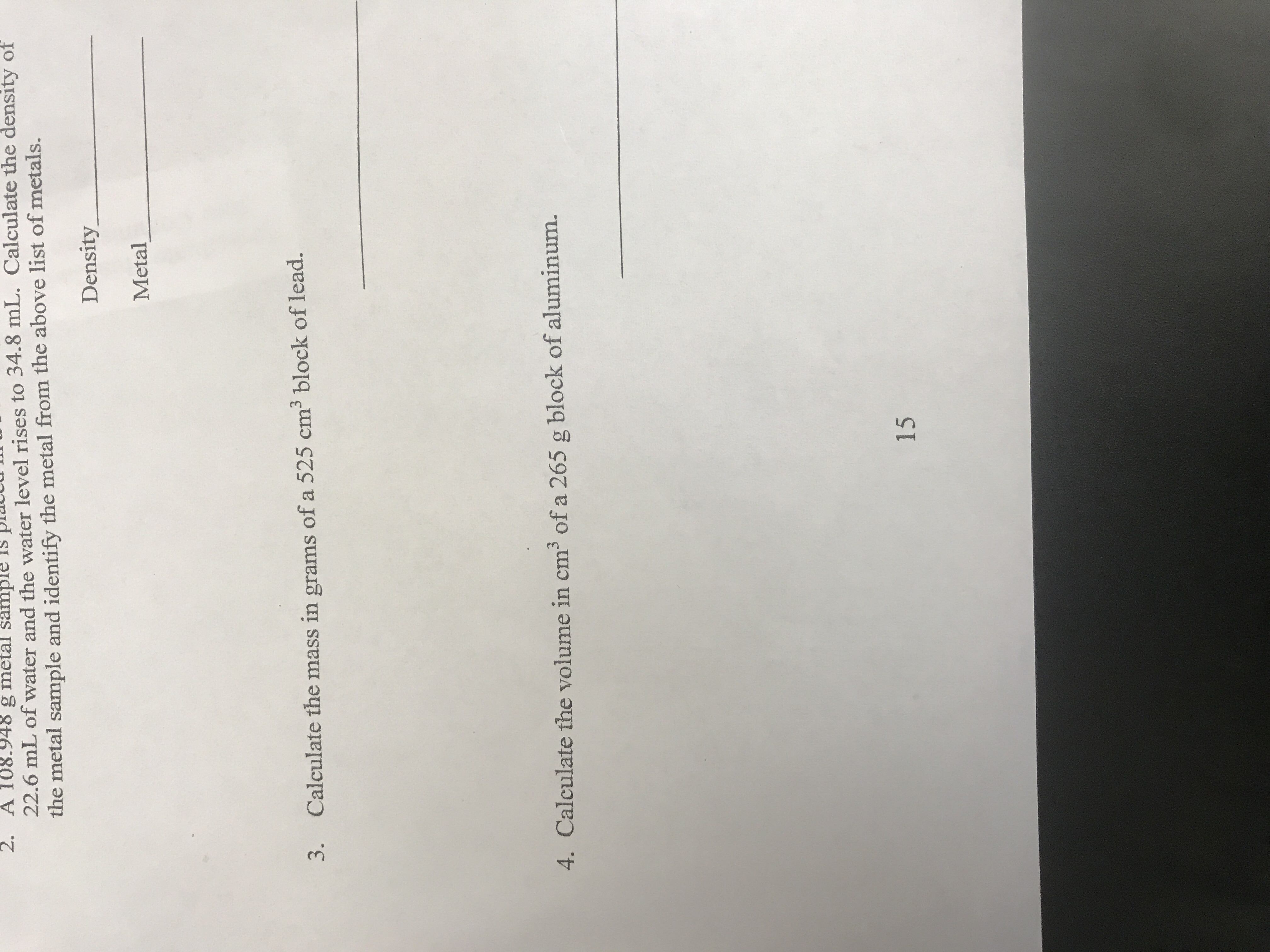# 2. A 108.948 g metal sample22.6 mL of water and the water level rises to 34.8 mL. Calculate the density ofthe metal sample and identify the metal from the above list of metals.DensityMetal33.Calculate the mass in grams of a 525 cm block of lead.4. Calculate the volume in cm3 of a 265 g block of aluminum.15

Question
1 viewshelp_outlineImage Transcriptionclose2. A 108.948 g metal sample 22.6 mL of water and the water level rises to 34.8 mL. Calculate the density of the metal sample and identify the metal from the above list of metals. Density Metal 3 3. Calculate the mass in grams of a 525 cm block of lead. 4. Calculate the volume in cm3 of a 265 g block of aluminum. 15 fullscreen
check_circle

Step 1

We’ll answer the first question since the exact one wasn’t specified. Please submit a new question specifying the one you’d like answered. Since question-2 is not clear in image so question-3 solution is given.

Step 2

Density is a physical quantity that can be determined with the help of the mass and volume of the given substance.

Step 3

The formula of the density of any s...

### Want to see the full answer?

See Solution

#### Want to see this answer and more?

Solutions are written by subject experts who are available 24/7. Questions are typically answered within 1 hour.*

See Solution
*Response times may vary by subject and question.
Tagged in

### Chemistry